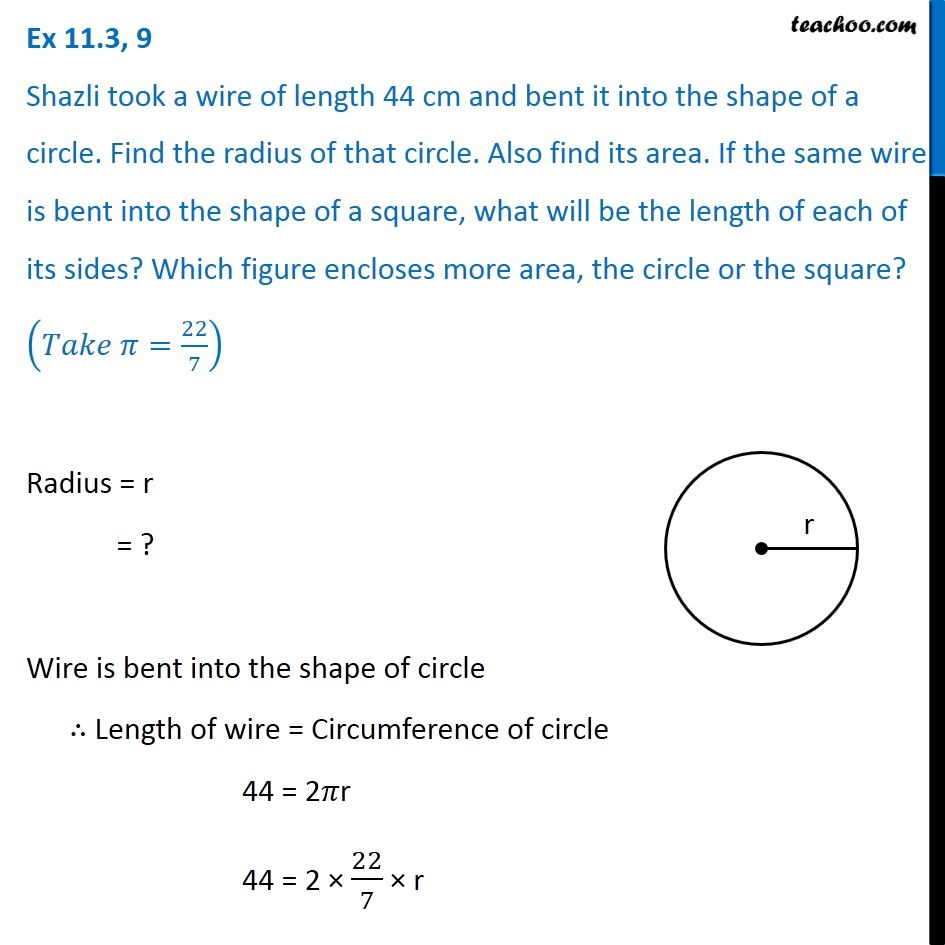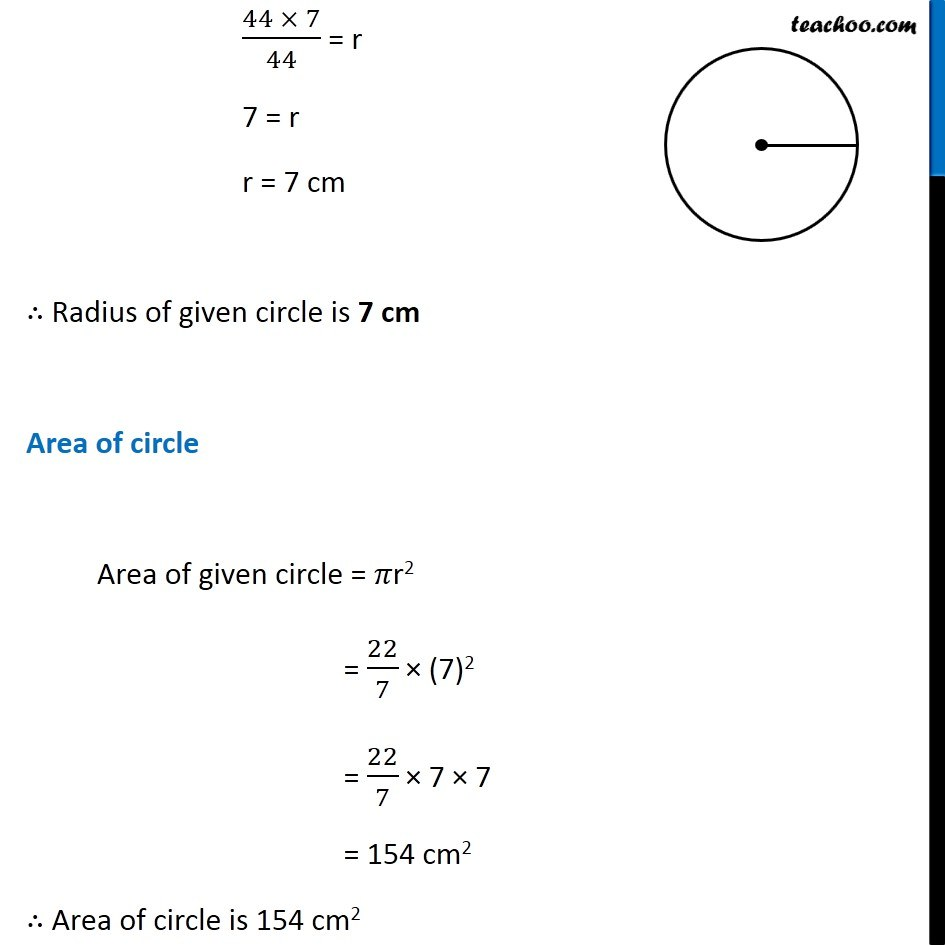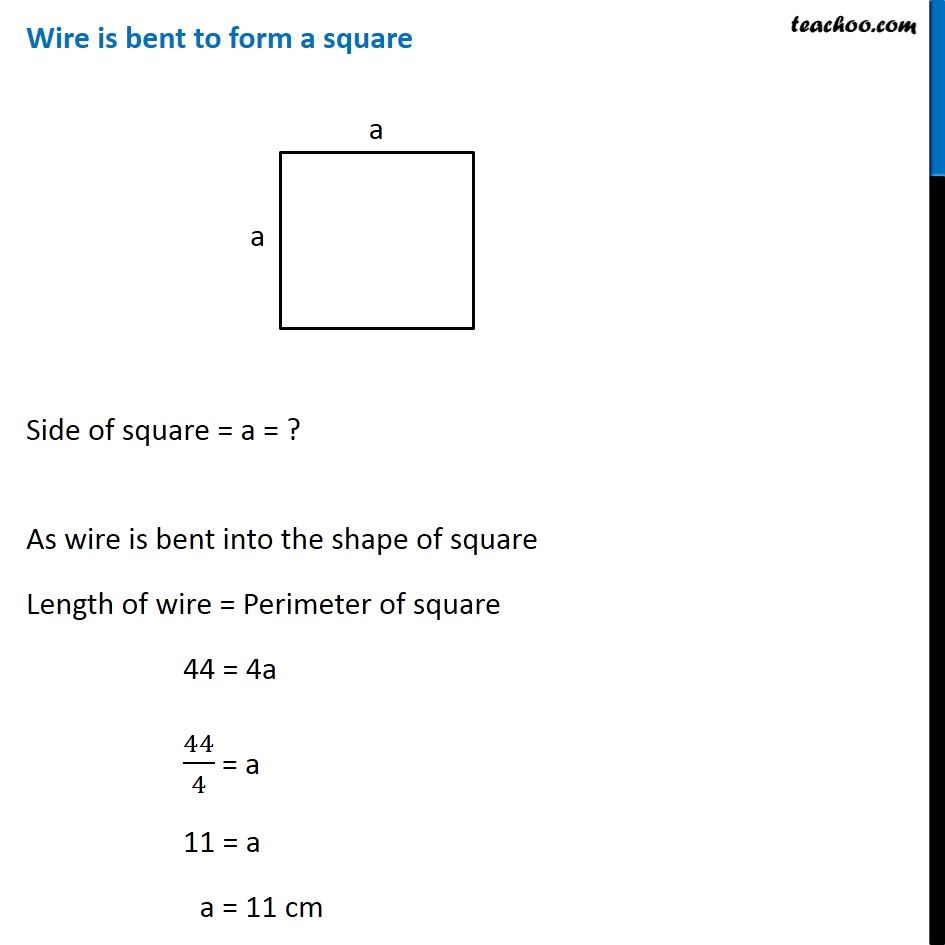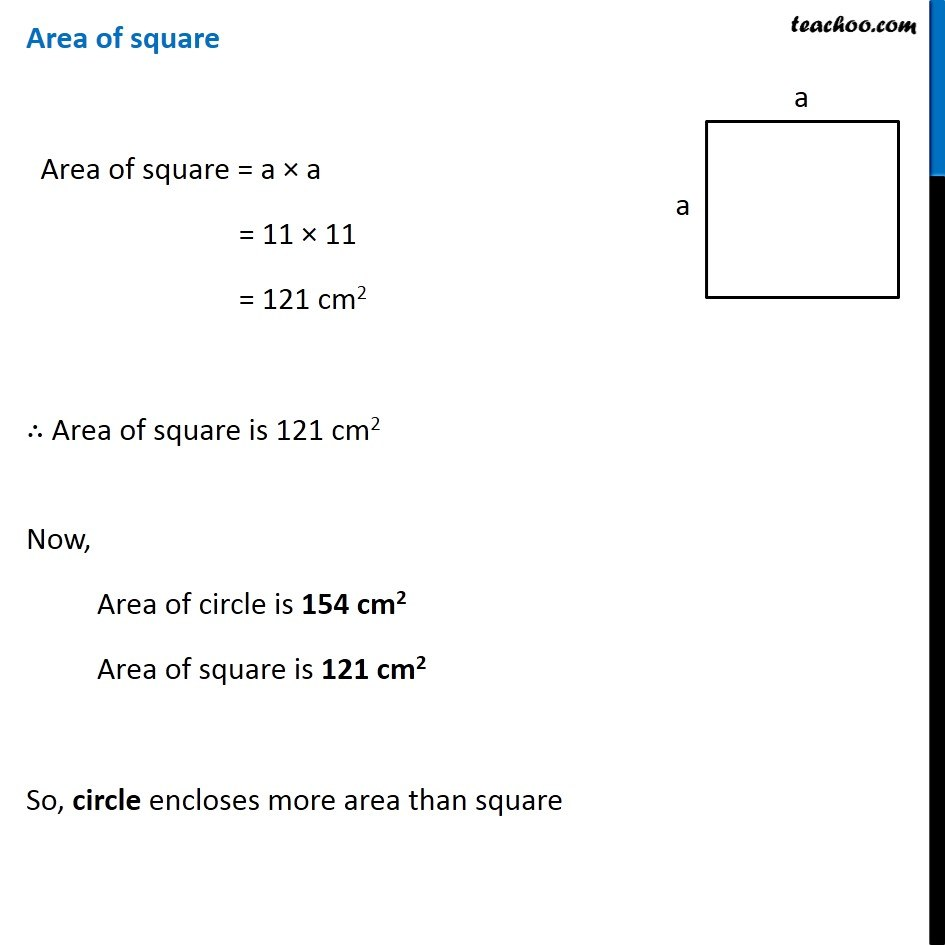Area of combination of figures - two circles, circle and square

Chapter 9 Class 7 Perimeter and Area
Concept wiseGet live Maths 1-on-1 Classs - Class 6 to 12

### Transcript

Ex 9.2, 9 Shazli took a wire of length 44 cm and bent it into the shape of a circle. Find the radius of that circle. Also find its area. If the same wire is bent into the shape of a square, what will be the length of each of its sides? Which figure encloses more area, the circle or the square? (𝑇𝑎𝑘𝑒 𝜋=22/7) Radius = r = ? Wire is bent into the shape of circle ∴ Length of wire = Circumference of circle 44 = 2𝜋r 44 = 2 × 22/7 × r (44 × 7)/44 = r 7 = r r = 7 cm ∴ Radius of given circle is 7 cm Area of circle Area of given circle = 𝜋r2 = 22/7 × (7)2 = 22/7 × 7 × 7 = 154 cm2 ∴ Area of circle is 154 cm2 Wire is bent to form a square Side of square = a = ? As wire is bent into the shape of square Length of wire = Perimeter of square 44 = 4a 44/4 = a 11 = a a = 11 cm Area of square Area of square = a × a = 11 × 11 = 121 cm2 ∴ Area of square is 121 cm2 Now, Area of circle is 154 cm2 Area of square is 121 cm2 So, circle encloses more area than square